Next: H.2 Byte Patterns Up: H.1 Basic Formats Previous: H.1.1 Single

## H.1.2 Double

A 64-bit double format number X is divided as shown in Fig. H.2. The value v of X is inferred from its constituent fields thus

1.
If e = 2047 and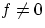, then v is NaN regardless of s
2.
If e = 2047 and f = 0, then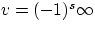3.
If 0 < e < 2047, then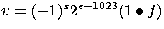4.
If e = 0 and, then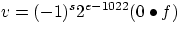(denormalized numbers)
5.
If e = 0 and f = 0, then v = (-1)s0 (zero)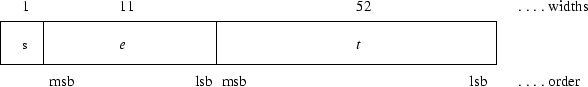5/13/1999